# RD Sharma Class 8 Solutions Chapter 1 Rational Numbers Ex 1.7

In this chapter, we provide RD Sharma Class 8 Solutions Chapter 1 Rational Numbers Ex 1.7 for English medium students, Which will very helpful for every student in their exams. Students can download the latest RD Sharma Class 8 Solutions Chapter 1 Rational Numbers Ex 1.7 Maths pdf, free RD Sharma Class 8 Solutions Chapter 1 Rational Numbers Ex 1.7 Maths book pdf download. Now you will get step by step solution to each question.

### RD Sharma Solutions for Class 8 Chapter 1 Rational Numbers Ex 1.7 Download PDF

Question 1.
Divide :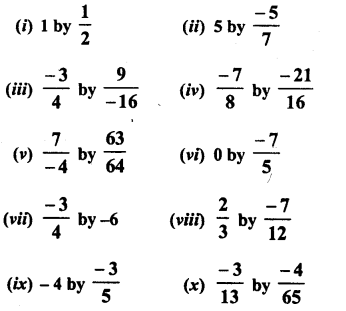Solution: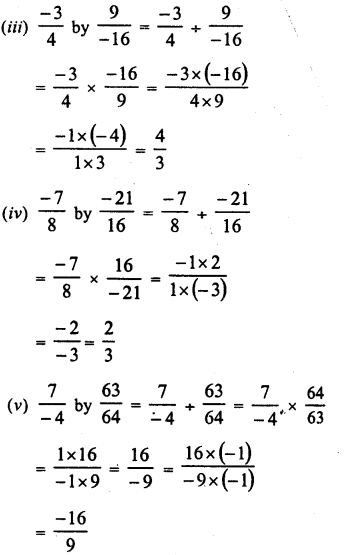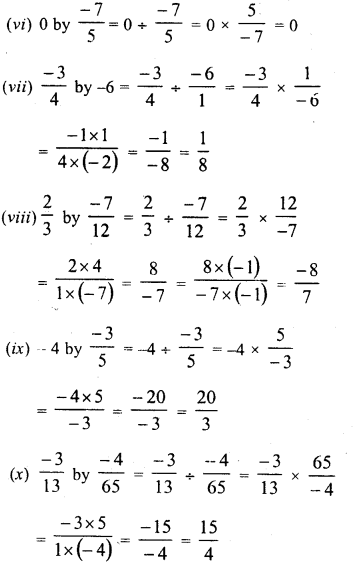Question 2.
Find the value and express as a rational number in standard form :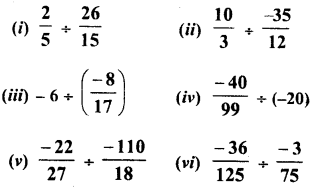Solution:Question 3.
The product of two rational numbers is15. If one of the numbers is -10, find the other.
Solution:Question 4.
The product of two rational numbers is−98 if one of the numbers is −415, find other.
Solution:Question 5.
By what number should we multiply −16 so that the product may be −239 ?
Solution: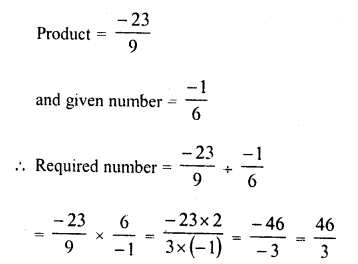Question 6.
By what number should we multiply −1528 so that the product may be −57 ?
Solution: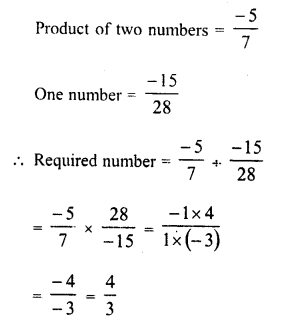Question 7.
By what number should we multiply −813 so that the product may be 24 ?
Solution: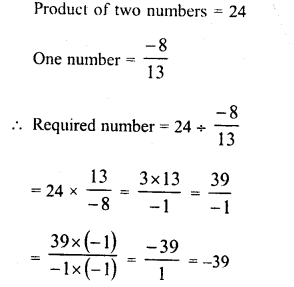Question 8.
Bv what number should −34 multiplied in order to produce 23 ?
Solution:Question 9.
Find (x +y) + (x – y), if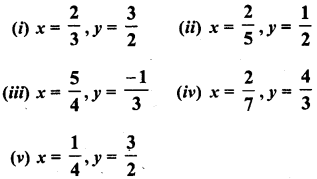Solution: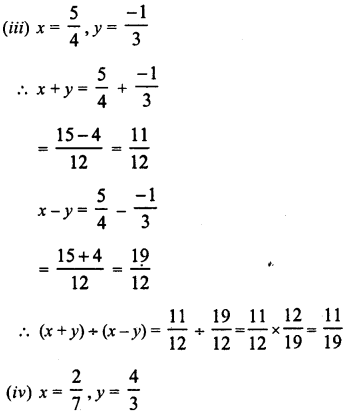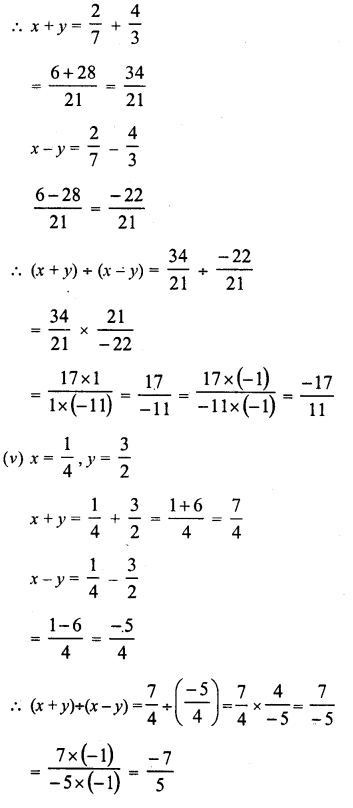Question 10.
The cost of 7 23 metres of rope is Rs 12 34.Find its cost per metre.
Solution:Question 11.
The cost of 2 13 metres of cloth is Rs. 75 14Find the cost of cloth per metre.
Solution:Question 12.
By what number should −3316 be divided to get −114 ?
Solution:Question 13.
Divide the sum of −135 and 127 by the product of −317 and −12.
Solution: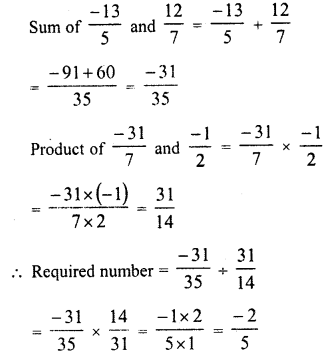Question 14.
Divide the sum of 6512 and 127 bv their difference.
Solution: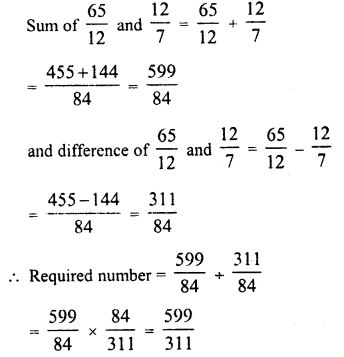Question 15.
If 24 trousers of equal size can be prepared in 54 metres of cloth, what length of cloth is required for each trouser ?
Solution: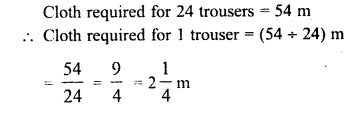All Chapter RD Sharma Solutions For Class 8 Maths

—————————————————————————–

All Subject NCERT Exemplar Problems Solutions For Class 8

All Subject NCERT Solutions For Class 8

*************************************************

I think you got complete solutions for this chapter. If You have any queries regarding this chapter, please comment on the below section our subject teacher will answer you. We tried our best to give complete solutions so you got good marks in your exam.

If these solutions have helped you, you can also share rdsharmasolutions.in to your friends.